# 1 Intro

## 1.1 Purpose

The function `soundgen` is intended for the synthesis of animal vocalizations, including human non-linguistic vocalizations like sighs, moans, screams, etc. It can also create non-biological sounds that require precise control over spectral and temporal modulations, such as special sound effects in computer games or acoustic stimuli for scientific experiments. Soundgen is NOT meant to be used for text-to-speech conversion. It can be adapted for this purpose, but existing specialized tools will probably serve better.

Soundgen uses a parametric algorithm, which means that sounds are synthesized de novo, and the output is completely determined by the values of control parameters, as opposed to concatenating or modifying existing audio recordings. Under the hood, the current version of soundgen generates and filters two sources of excitation: sine waves and white noise.

The rest of this vignette will unpack this last statement and demonstrate how soundgen can be used in practice. To simplify setting the control parameters and visualizing the output, soundgen library includes an interactive Shiny app. To start the app, type `soundgen_app()` from R or try it online at cogsci.se/soundgen.html. To generate sounds from the console, use the function `soundgen`. Each section of the vignette focuses on a particular aspect of sound generation, both describing the relevant arguments of `soundgen` and explaining how they can be set in the Shiny app. Note that some advanced features, notably vectorization of several arguments, are not implemented in the app and are only accessible from the console.

TIP: this vignette is a hands-on, non-technical tutorial focusing on how to use soundgen in order to synthesize new sounds. For a more rigorous and theoretical discussion, please refer to Anikin, A. (2019). Soundgen: an open-source tool for synthesizing nonverbal vocalizations. Behavoir Research Methods, 51(2), 778-792.

## 1.2 Before you proceed: consider the alternatives

There are several other R packages that offer sound synthesis, notably `tuneR`, `seewave`, and `phonTools`. Both `seewave` and `tuneR` implement straightforward ways to synthesize pulses and square, triangular, or sine waves as well as noise with adjustable (linear) spectral slope. You can also create multiple harmonics with both amplitude and frequency modulation using `seewave::synth()` and `seewave::synth2()`. There is even a function available for adding formants and thus creating different vowels: `phonTools::vowelsynth()`. Basic tonal synthesis and many acoustic manipulations can also be performed using the open-source program PRAAT. If this is ample for your needs, you might want to try these alternatives first.

So why bother with soundgen? First, it takes customization and flexibility of sound synthesis much further. You will appreciate this flexibility if your aim is to produce convincing biological sounds. And second, it’s a higher-level tool with dedicated subroutines for things like controlling the rolloff (relative energy of different harmonics), adding moving formants and antiformants, mixing harmonic and noise components, controlling voice changes over multiple syllables, adding stochasticity to imitate unpredictable voice changes common in biological sound production, and more. In other words, soundgen offers powerful control over low-level acoustic characteristics of synthesized sounds with the benefit of also offering transparent, meaningful high-level parameters intended for rapid and straightforward specification of whole bouts of vocalizing.

Because of this high-level control, you don’t really have to think about the math of sound synthesis in order to use soundgen (although if you do, that helps). This vignette also assumes that the reader has some training in phonetics or bioacoustics, particularly for sections on formants and subharmonics.

## 1.3 Basic principles of sound synthesis in soundgen

Feel free to skip this section if you are only interested in using soundgen, not in how it works under the hood.

Soundgen’s credo is to start with a few control parameters (e.g., the intonation contour, the amount of noise, the number of syllables and their duration, etc.) and to generate a corresponding audio stream, which will sound like a biological vocalization (a bark, a laugh, etc). The core algorithm for generating a single voiced segment implements the standard source-filter model (Fant, 1971). The voiced component is generated as a sum of sine waves and the noise component as filtered white noise, and both components are then passed through a frequency filter simulating the effect of human vocal tract. This process can be conceptually divided into three stages:

1. Generation of the harmonic component (glottal source). At this crucial stage, we “paint” the spectrogram of the glottal source based on the desired intonation contour and spectral envelope by specifying the frequencies, phases, and amplitudes of a number of sine waves, one for each harmonic of the fundamental frequency (f0). If needed, we also add stochastic and non-linear effects at this stage: jitter and shimmer (random fluctuation in frequency and amplitude), subharmonics, slower random drift of control parameters, etc. Once the spectrogram “painting” is complete, we synthesize the corresponding waveform by generating and adding up as many sine waves as there are harmonics in the spectrum.

Note that soundgen currently implements only sine wave synthesis of voiced fragments. This is different from modeling glottal cycles themselves, as in phonetic models and some popular text-to-speech engines (e.g. Klatt, 1980). Normally multiple glottal cycles are generated simultaneously, with no pauses in between them (no closed phase) and with a continuously changing f0. It is also possible to add a closed phase, in which case each glottal cycle is generated separately, with f0 held stable within each cycle. In future versions of soundgen there may be an option to use a particular parametric model of the glottal cycle as excitation source as an alternative to generating a separate sine wave for each harmonic.

1. Generation of the turbulent noise component (aspiration, hissing, etc.). In addition to harmonic oscillations of the vocal cords, there are other sources of excitation, notably turbulent noise. For example, aspiration noise may be synthesized as white noise and added to the glottal source before formant filtering. It is similarly straightforward to add other types of noise, which may originate higher up in the vocal tract and thus display a different formant structure from the glottal source (e.g., high-frequency hissing, broadband clicks for tongue smacking, etc.)

Some form of noise is synthesized in most sound generators. In soundgen noise is created in the frequency domain (i.e., as a spectrogram) and then converted into a time series via inverse FFT. Noise is generated with a flat spectrum up to a certain threshold, followed by user-specified linear rolloff (Johnson, 2012).

1. Spectral filtering (formants and lip radiation). The vocal tract acts as a resonator that modifies the source spectrum by amplifying certain frequencies and dampening others. In speech, time-varying resonance frequencies (formants) are responsible for the distinctions between different vowels, but formants are also ubiquitous in animal vocalizations. Just as we “painted” a spectrogram for the acoustic source in (1), we now “paint” a spectral filter with a specified number of stationary or moving formants. We then take a short-time Fourier transform (STFT) of the generated waveform to convert it back to a spectrogram, multiply the latter by our filter, and then take an inverse STFT to go back to the time domain. This filtering can be applied to harmonic and noise components separately or - for noise sources close to the glottis - the harmonic component and the noise component can be added first and then filtered together.

Note that this STFT-mediated method of adding formants is different from the more traditional convolution, but with multiple formants it is both considerably faster and (arguably) more intuitive. If you are wondering why we don’t simply apply the filter to the rolloff matrix before the iSTFT, this is an annoying consequence of some complexities of the temporal structure of a bout, especially of applying non-stationary filters (moving formants) that span multiple syllables. For the noise component, however, this extra step can be avoided, and we only do iSTFT once.

Having briefly looked at the fundamental principles of sound generation, we proceed to control parameters. The aim of the following presentation is to offer practical tips on using soundgen. For further information on more fundamental principles of acoustics and sound synthesis, you may find the vignettes in `seewave` very helpful, or you can check out the book on sound synthesis in R by Jerome Sueur, the author of the `seewave` package. Some essential references are also listed at the end of this vignette, especially those sources that have inspired particular routines in soundgen.

# 2 Using soundgen

## 2.1 Where to start

To generate a sound, you can either type `soundgen_app()` to open an interactive Shiny app or call `soundgen()` from R console with manually specified parameters. The app offers nice visualizations and is more user-friendly if you are not used to programming, but note that it doesn’t support some advanced features (e.g., vectorization of some control parameters). An object called `presets` contains a collection of presets that demonstrate some of the possibilities. More information is available on the project’s homepage at http://cogsci.se/soundgen.html.

## 2.2 Audio playback

Audio playback may fail, depending on your platform and installed software. Soundgen relies on `tuneR` library for audio playback, via a wrapper function called `playme()` that accepts both Wave objects and simple numeric vectors. If `soundgen(play = TRUE)` throws an error, make sure the audio can be played before you proceed with using soundgen. To do so, save some sound as a vector first: `sound = soundgen(play = FALSE)` or even simply `sound = rnorm(10000)`. Then try to find a way to play this vector `sound`. You may need to change the default player in `tuneR` or install additional software. See the seewave vignette on sound input/output for an in-depth discussion of audio playback in R. Sueur (2018, p. 100) recommends Windows Media Player on Windows, AudioUnits for Mac OS, SoX for Linux (the player is called “play”), or VLC on any platform. I find that “play” from the “vox” library or “aplay” work well on Linux, and “afplay” on Macs.

Because of possible errors, audio playback is disabled by default in the rest of this vignette. To turn it on without changing any code, simply set the global variable `playback` to the appropriate value for your specific OS, for ex.:

``````playback = list(TRUE, FALSE, 'vlc', 'my-awesome-player')[]
# TRUE means defaulting to "play" on Linux, "afplay" on Mac,
# and the defaults of tuneR::play on Windows
# FALSE means no sound playback``````

## 2.3 From the console

The basic workflow from R console is as follows:

``````library(soundgen)
s001 = soundgen(play = playback)  # default sound: a short [a] by a male speaker
# 's' is a numeric vector - the waveform. You can save it, play it, plot it, ...``````
``````# names(presets)  # speakers in the preset library
# names(presets\$Chimpanzee)  # presets per speaker
s002 = eval(parse(text = presets\$Chimpanzee\$Scream_conflict))  # a screaming chimp
# playme(s)``````

## 2.4 From the app

The basic workflow in the Shiny app is as follows:

1. Start the app by typing `soundgen_app()`. RStudio should open it in the default web browser (there will be no sound if the app runs in an RStudio window instead of a browser). Firefox and Chrome are known to work. Safari will probably fail to play back the generated audio, although the output can still be exported as a .wav file.
2. Set parameters in the tabs on the left (see the sections below for details). You can also start with a preset that resembles the sound you want and then fine-tune control parameters.
3. Check the preview plots and tables of anchors to ensure you get what you want.
4. Click Generate. This will create a .wav file, play it, and display the spectrogram or long-term average spectrum.
5. Save the generated sound or go back to (1) to make further adjustments.

TIP The interactive app `soundgen_app()` gives you the exact R code for calling `soundgen()`, which you can copy-paste into your R environment and generate manually the same sound as the one you have created in the app. If in doubt about the right format for a particular argument, you can use the app first, copy-paste the code into your R console, and modify it as needed. You can also import an existing formula into the app, adjust the parameters in an interactive environment, and then export it again. BUT: the app can only use a single value for many parameters that are vectorized when called from the command line (rolloff, jitterDep, etc.).

## 2.5 Syllables

If you need to generate a single syllable without pauses, the only temporal parameter you have to set is `sylLen` (“Syllable length, ms” in the app). For a bout of several syllables, you have two options:

1. Set `nSyl` (“Number of syllables” in the app). Unvoiced noise is then allowed to fill in the pauses (if noise is longer than the voiced part), and you can specify an amplitude contour, intonation contour, and formant transitions that will span the entire bout. For ex., if the vowel sequence in a three-syllable bout is “uai”, the output will be approximately “[u] – pause – [a] – pause – [i]”.
``````s003 = soundgen(formants = 'uai', repeatBout = 1, nSyl = 3, play = playback)
# to replay without re-generating the sound, type "playme(s)"``````
1. Set `repeatBout` (“Repeat bout # times” in the app). This is the same as calling `soundgen` repeatedly with the same settings or clicking the Generate button in the app several times. If temperature = 0, you will get exactly the same sound repeated each time, otherwise some variation will be introduced. For the same “uai” example, the output will be “[uai] – pause – [uai] – pause – [uai]”.
``````s004 = soundgen(formants = 'uai', repeatBout = 3, nSyl = 1, play = playback)
# playme(s)``````

Like most arguments to soundgen, `sylLen` and `pauseLen` can also be vectors. For example, if you want to synthesize 5 syllables of progressively shorter duration and separated by increasingly longer pauses, you can write:

``````s005 = soundgen(nSyl = 5,
sylLen = c(300, 100),   # linearly decreasing from 300 to 100 ms
pauseLen = c(50, 150),  # increasing from 50 to 150 ms
plot = TRUE,
play = playback)``````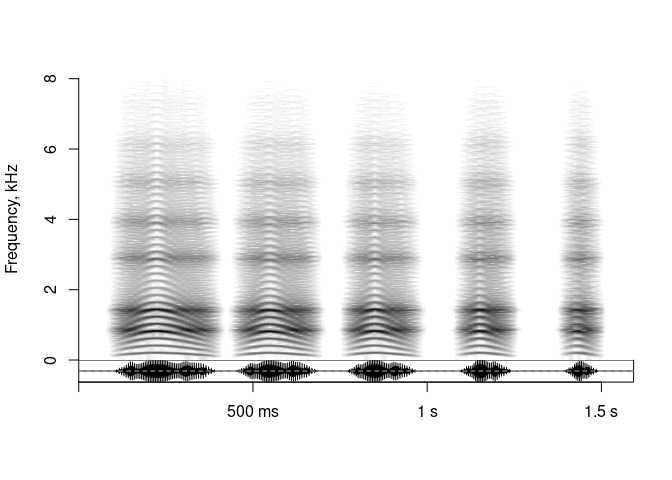``# playme(s)``

For more complicated changes in the length of syllables or pauses, you can use the function `getSmoothContour` to upsample your anchors (see “Intonation” for examples) or manually code longer sequences of values. The length of your input vector doesn’t matter: it will be up- or downsampled automatically. This also works with all other vectorized arguments to soundgen (rolloff, jitterDep, vibratoFreq, etc).

``````s006 = soundgen(
nSyl = 10,
sylLen = c(60, 200, 90, 50, 50),  # quickly up to 200 and down to 50
pauseLen = c(50, 60, 80, 150),    # growing ~exponentially
plot = TRUE,
play = playback
)``````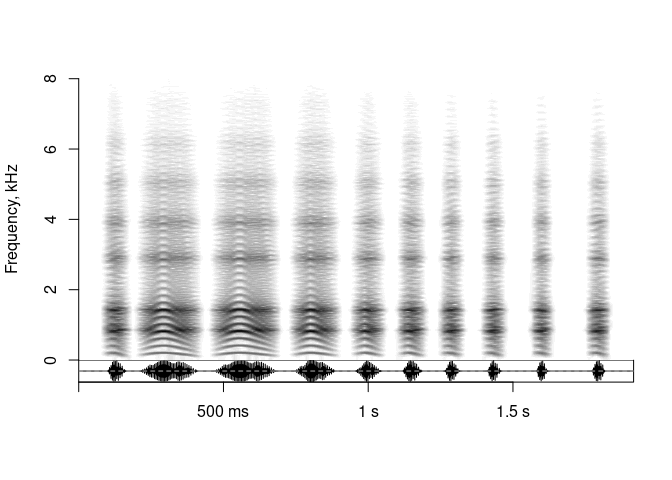As a special case, your values will be used without interpolation if you provide exactly as many as needed:

``````s007 = soundgen(
nSyl = 5,
sylLen = c(300, 100, 400, 50, 100),  # 5 syllables, 5 values
pauseLen = c(50, 150, 50, 100),      # 4 pauses, 4 values
plot = TRUE,
play = playback
)``````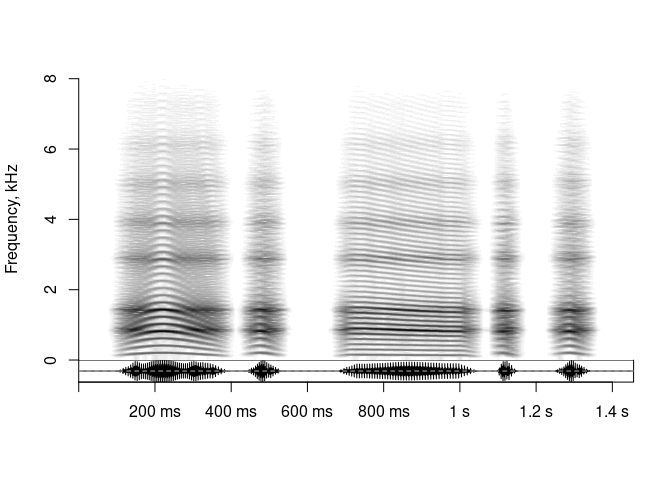You can use both `repeatBout` and `nSyl` simultaneously. The pause between bouts is equal to the length of the first syllable:

``````s008 = soundgen(
repeatBout = 2,
nSyl = 3,
sylLen = c(300, 100),
pauseLen = c(100, 50),
plot = TRUE,
play = playback
)``````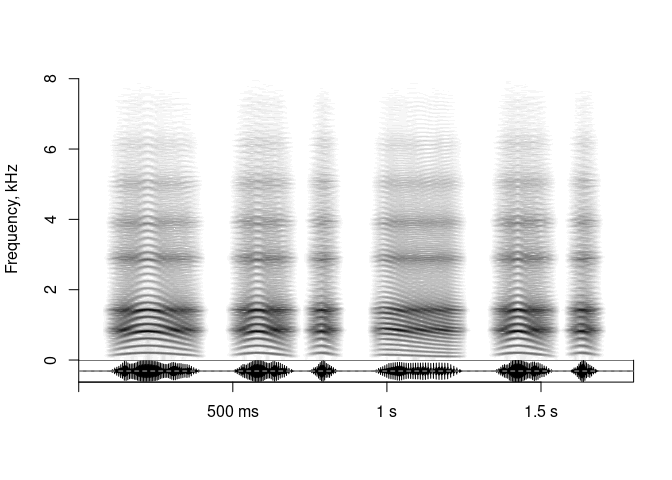Note that all pauses between syllables have to be positive. A negative pause (overlap) between bouts is allowed, but you have to enforce it with `invalidArgAction = "ignore"`:

``````s009 = soundgen(
repeatBout = 2,
sylLen = c(300, 100),
pauseLen = -50,
plot = TRUE,
play = playback,
invalidArgAction = 'ignore'
)``````
``````## Warning in validatePars(p, gp, permittedValues, invalidArgAction):
## pauseLen should be between 0 and 1000; override with caution``````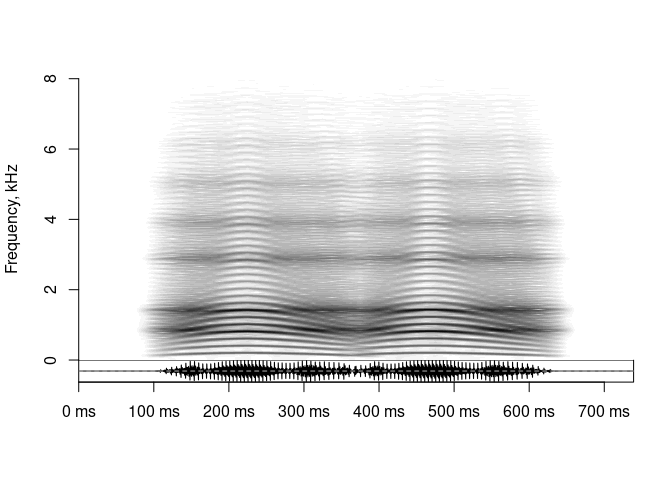## 2.6 Intonation

### 2.6.1 One syllable

When we hear a tonal sound such as someone singing, one of its most salient characteristics is intonation or, more precisely, the contour of the fundamental frequency (f0), or, even more precisely, the contour of the physically present or perceptually extrapolated spectral band which is perceived to correspond to the fundamental frequency (pitch). Soundgen literally generates a sine wave corresponding to f0 and several more sine waves corresponding to higher harmonics, so f0 is straightforward to implement. However, how can its contour be specified with as few parameters as possible? The solution adopted in soundgen is to take one or more anchors as input and generate a smooth contour that passes through all anchors.

In the simplest case, all anchors are equidistant, dividing the sound into equal time steps. You can then specify anchors as a numeric vector. For example:

``````# steady pitch at 440 Hz
s010 = soundgen(pitch = 440, play = playback) ``````
``````# downward chirp
s011 = soundgen(pitch = 3000:2000, play = playback,
samplingRate = 44100, pitchSamplingRate = 44100)
# when f0 is high, increase samplingRate and pitchSamplingRate for better quality``````
``````# up and down
s012 = soundgen(pitch = c(150, 250, 100), sylLen = 700, play = playback) ``````
``````# 3rd quarter silent
s013 = soundgen(pitch = c(150, 200, NA, 110),
sylLen = 700, play = playback)  ``````

You can also use a mathematical formula to produce very precise pitch modulation, just check that the values are on the right scale. For example, sinusoidal pitch modulation can be created as follows:

``````anchors = (sin(1:70 / 3) * .25 + 1) * 350
plot(anchors, type = 'l', xlab = 'Time (points)', ylab = 'Pitch (Hz)')
s014 = soundgen(pitch = anchors, sylLen = 1000, play = playback)``````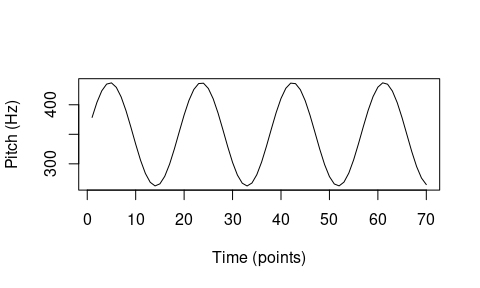For more flexibility, anchors can also be specified at arbitary times using the “anchor format” - a dataframe with two columns: `time` (ms) and `value` (in the case of pitch, this is frequency in Hz). The function that generates smooth contours of f0 and other parameters is `getSmoothContour()`. When you generate sounds, `soundgen()` has an argument `smooth(list = ...)`, where you can put the settings passed on to `getSmoothContour()`. So you do not have to call `getSmoothContour()` explicitly, although sometimes it can be helpful to do so in order to visualize the curve implied by your anchors. Time can range from 0 to 1, or it can be specified in ms – it makes no difference, since the produced contour is rescaled to match syllable duration.

For example, say we want f0 first to increase sharply from 350 to 700 Hz and then to slowly return to baseline. Time anchors can then be specified as `c(0, .1, 1)` (think of it as “start”, “10%”, and “end” of the sound), and the arguments `len` and `samplingRate` together determine the duration: `len / samplingRate` gives duration in seconds. Values are processed on a logarithmic (musical) scale if `thisIsPitch` is `TRUE`, and the resulting curve is smoothed (the default behavior is to use loess for up to 10 anchors, cubic spline for 11-50 anchors, and linear interpolation for >50 anchors).

A sound with this intonation can be generated as follows:

``````s015 = soundgen(
sylLen = 900, play = playback,
pitch = list(time = c(0, .1, 1),  # or (c(0, 30, 300)) - in ms
value = c(350, 700, 350)))``````

Beware of smoothing! A curve interpolated from a few anchors is not uniquely defined, and the interpolation algorithm has a major effect on its shape. The amount of smoothing can be controlled with `loessSpan` and `interpol`:

``````sylLen = 500  # desired syllable length, in ms
samplingRate = 16000
sylLen_points = sylLen / 1000 * samplingRate
anchors = data.frame(time = c(0, .1, 1),
value = c(350, 700, 350))

par(mfrow = c(1, 3))
smc1 = getSmoothContour(
anchors = anchors,
len = sylLen_points,
interpol = 'approx',
thisIsPitch = TRUE, plot = TRUE,
main = 'No smoothing', samplingRate = samplingRate
)
sm2 = getSmoothContour(
anchors = anchors,
len = sylLen_points,
loessSpan = 0.75,
thisIsPitch = TRUE, plot = TRUE,
main = 'loessSpan = .75', samplingRate = samplingRate
)
smc3 = getSmoothContour(
anchors = anchors,
len = sylLen_points,
loessSpan = 1,
thisIsPitch = TRUE, plot = TRUE,
main = 'loessSpan = 1', samplingRate = samplingRate
)
par(mfrow = c(1, 1))
# likewise: soundgen(smoothing = list(interpol = 'loess', loessSpan = 1))``````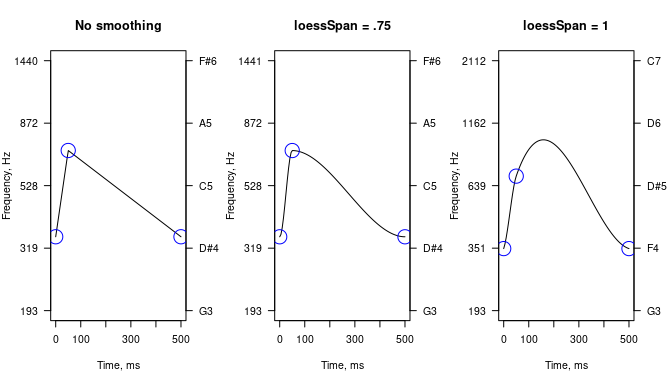If you are not satisfied with the smooth curve generated by `soundgen()` based on your anchors, you can produce a longer vector (e.g., you could use `analyze()` or `pitch_app()` to extract the pitch contour of an existing recording), and then you can feed soundgen with this arbitrarily long vector instead of using the anchor format, ensuring very precise control over the intonation contour.

TIP Many arguments to soundgen are vectorized, and most vectorized arguments understand the “anchor format” you just encountered above, namely something like `my_argument = data.frame(time = ..., value = ...)`, where time can be in ms or ~[0, 1]. See ?soundgen for a complete list of anchor-format arguments and keep in mind two important special cases that use a slightly different format: `formants` and `noise` (see below). And remember to check that interpolation looks reasonable!

To get more complex curves, simply add more anchors. The assumption behind specifying an entire contour with a few discrete anchors is that the contour is smooth and continuous. However, there may be special occasions when you do want a discontinuity such as an instantaneous pitch jump. The default behavior of `getSmoothContour()` is to make a jump if two anchors are closer than one percent of the syllable length (as specified with the default `jumpThres = 0.01`). To make a pitch jump, you thus provide two values of f0 that are very close in time, for example:

``````s016 = soundgen(sylLen = 800, plot = TRUE, play = playback,
pitch = list(time = c(0, .2, .201, .4, 1),
value = c(900, 1200, 1800, 2000, 1500)),
samplingRate = 22050)``````
``## pitchSampingRate should be much higher than the highest pitch; resetting to 20000 Hz``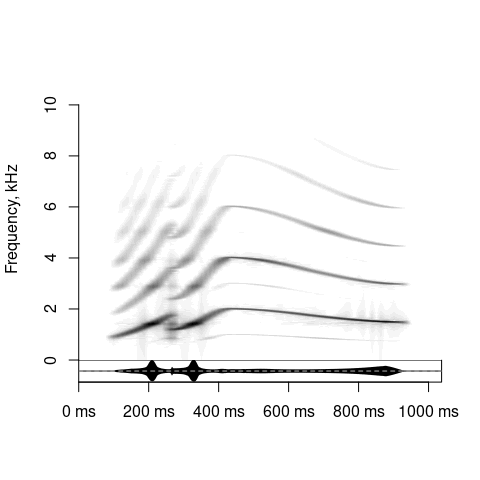TIP Given the same anchors, the shape of the resulting curve depends on syllable duration. That’s because the amount of smoothing is adjusted automatically as you change syllable duration. Double-check that all your contours still look reasonable if you change the duration!

To draw f0 contour in the Shiny app, use “Intonation / Intonation syllable” tab and click the intonation plot to add anchors. Soundgen then generates a smooth curve through these anchors. If you click the plot close to an existing anchor, the anchor moves to where you clicked; if you click far from any existing anchor, a new anchor is added. To remove an anchor, double-click it. To go back to a straight line, click the button labeled “Flatten pitch contour”. Exactly the same principles apply to all anchors in soundgen_app (pitch, amplitude, mouth opening, and noise). Note also that all contours are rescaled when the duration changes, with the single exception of negative time anchors for noise (i.e. the length of pre-syllable aspiration does not depend on syllable duration).

### 2.6.2 Multiple syllables

If the bout consists of several syllables (`nSyl > 1`), you can also specify the overall intonation over several syllables using `pitchGlobal` (app: “Intonation / Intonation global”). The global intonation contour specifies the deviation of pitch per syllable from the main pitch contour in semitones, i.e. 12 semitones = 1 octave. In other words, it shows how much higher or lower the average pitch of each syllable is compared to the rest of the syllables. For ex., we can generate five seagull-like sounds, which have the same intonation contour within each syllable, but which vary in average pitch spanning about an octave in an inverted U-shaped curve. Note that the number of anchors need not equal the number of syllables:

``````s017 = soundgen(nSyl = 5, sylLen = 200, pauseLen = 140,
plot = TRUE, play = playback,
pitch = list(time = c(0, 0.65, 1),
value = c(977, 1540, 826)),
pitchGlobal = list(time = c(0, .5, 1),
value = c(-6, 7, 0)))``````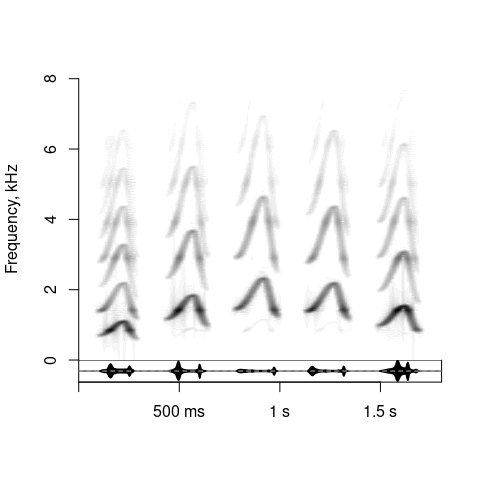``# pitchGlobal = c(-6, 7, 0) is equivalent, since time steps are equal``

TIP Calling `soundgen` with argument `plot = TRUE` produces a spectrogram using a function from soundgen package, `spectrogram`. Type `?spectrogram` or `?spectrogramFolder` and see the vignette on acoustic analysis for plotting tips and advanced options. You can also plot the waveform produced by `soundgen` using any other function, e.g. `seewave::spectro`

### 2.6.3 Vibrato

Vibrato adds frequency modulation (FM) to f0 contour by modifying f0 per glottal cycle. In contrast to irregular jitter and temperature-related random drift, this FM is regular, namely sinusoidal:

``````# variable, but deterministic vibrato (same every time)
s018 = soundgen(vibratoDep = 0:3, vibratoFreq = 7:5,
sylLen = 2000, pitch = c(300, 280),
play = playback, plot = TRUE)``````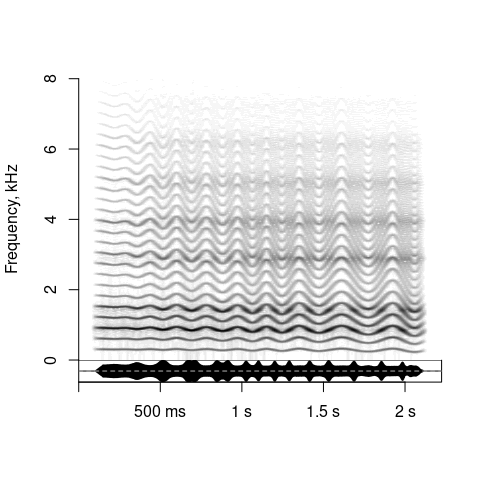``````# stochastic vibrato (different every time)
s019 = soundgen(vibratoDep = rnorm(n = 10, mean = .5, sd = .1),
vibratoFreq = rnorm(n = 10, mean = 5, sd = .5),
sylLen = 2000, pitch = c(300, 280),
play = playback, plot = TRUE)``````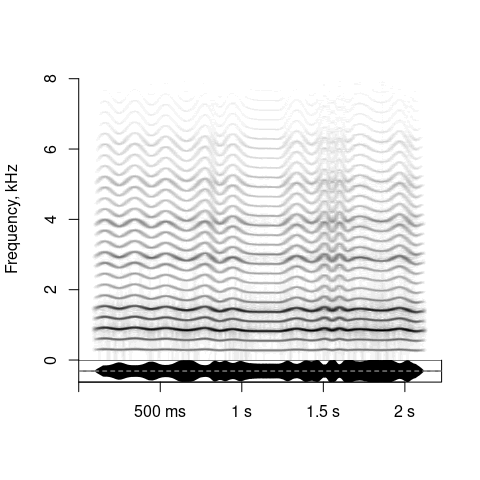## 2.7 Hyper-parameters

### 2.7.1 Temperature

It is a basic principle of soundgen that random variation can be introduced in the generated sound. This behavior is controlled by a single high-level parameter, `temperature` (app: “Main / Hypers”). If `temperature = 0`, you will get exactly the same sound by executing the same call to `soundgen` repeatedly. If `temperature > 0`, each generated sound will be somewhat different, even if all the control parameters are exactly the same. In particular, positive temperature introduces fluctuations in syllable structure, all contours (intonation, breathing, amplitude, mouth opening), and many effects (jitter, subharmonics, etc). It also “wiggles” user-specified formants and adds new formants above the specified ones at a distance calculated based on the estimated vocal tract length (see Section “Spectral filter (formants)” below).

Code example :

``````# the sound is a bit different each time, because temperature is above zero
s020 = soundgen(repeatBout = 5, temperature = 0.3, play = playback)
# Setting repeatBout = 5 is equivalent to:
# for (i in 1:5) soundgen(temperature = 0.3, play = playback)``````

If you don’t want stochastic behavior, set temperature to zero. But note that some effects, notably jitter and subharmonics, will then be added in an all-or-nothing manner: either to the entire sound or not at all. Also note that additional formants will not be added above the user-specified ones if temperature is exactly 0. In practice it may be better to set temperature to a very small positive value like 0.01. You can also change the extent to which temperature affects different parameters (e.g., if you want more variation in intonation and less variation in syllable structure). To do so, use `tempEffects`, which is a list of scaling coefficients that determine how much different parameters vary at a given temperature. `tempEffects` includes the following scaling coefficients:

• `amplDep`: random fluctuations of user-specified amplitude anchors across syllables (if nSyl > 1)
• `amplDriftDep`: drift of amplitude mirroring pitch drift
• `formDisp`: irregularity of the dispersion of stochastic formants that are added above user-specified formants (if any) at distances consistent with the specified length of the vocal tract `vocalTract`
• `formDrift`: the amount of random drift of formants
• `glottisDep`: proportion of glottal cycle with closed glottis
• `noiseDep`: random fluctuations of user-specified noise anchors across syllables (if nSyl > 1)
• `pitchDep`: random fluctuations of user-specified pitch anchors across syllables (if nSyl > 1)
• `pitchDriftDep`: amount of slow random drift of f0 (the higher, the more f0 changes)
• `pitchDriftFreq`: frequency of slow random drift of f0 (the higher, the faster f0 changes)
• `rolloffDriftDep`: drift of rolloff mirroring pitch drift
• `specDep`: random fluctuations of rolloff, nonlinear effects, attack
• `subDriftDep`: drift of subharmonic frequency and bandwidth mirroring pitch drift
• `sylLenDep`: random fluctuations of the duration of syllables and pauses between syllables

The default value of each scaling parameter is 1. To enhance a particular component of stochastic behavior, set the corresponding coefficient to a value >1; to remove it completely, set its scaling coefficient to zero.

``````# despite the high temperature, temporal structure does not vary at all,
# while formants are more variable than the default
s021 = soundgen(repeatBout = 3, nSyl = 2, temperature = .3, play = playback,
tempEffects = list(sylLenDep = 0, formDrift = 3))``````

### 2.7.2 Other hypers

To simplify usage, there are a few other hyper-parameters. They are redundant in the sense that they are not strictly necessary to produce the full range of sounds, but they provide convenient shortcuts by making it possible to control several low-level parameters at once in a coordinated manner. Hyper-parameters are marked “hyper” in the Shiny app.

For example, to imitate the effect of varying body size, you can use `maleFemale`. Since `formants` are not specified, but temperature is above zero, a schwa-like sound with approximately equidistant formants is generated using `vocalTract` (cm) to calculate the expected formant dispersion:

``````s022 = soundgen(
maleFemale = -1,  # male: 100% lower f0, 25% lower formants, 25% longer vocal tract
formants = NA, pitch = 220, vocalTract = 15, play = playback)
mf = c(-1,  # male: 100% lower f0, 25% lower formants, 25% longer vocal tract
0,   # neutral (default)
1)   # female: 100% higher f0, 25% higher formants, 25% shorter vocal tract
# See e.g. http://www.santiagobarreda.com/vignettes/v1/v1.html``````
``````s023 = soundgen(
maleFemale = 0,  # neutral (default)
formants = NA, pitch = 220, vocalTract = 15, play = playback)``````
``````s024 = soundgen(
maleFemale = 1,  # female: 100% higher f0, 25% higher formants, 25% shorter vocal tract
formants = NA, pitch = 220, vocalTract = 15, play = playback)``````

To change the basic voice quality along the breathy-creaky continuum, use `creakyBreathy`. It affects the rolloff of harmonics, the type and strength of pitch effects (jitter, subharmonics), and the amount of aspiration noise. For example:

``````cb = c(-1,  # max creaky
-.5, # moderately creaky
0,   # neutral (default)
.5,  # moderately breathy
1)   # max breathy (no tonal component)
silence = rep(0, 1600)
s025 = silence
for (i in cb) {
s025 = c(s025, soundgen(creakyBreathy = i), silence)
}
# playme(s025)``````

## 2.8 Amplitude envelope

Use `ampl` and `amplGlobal` to modulate the amplitude (loudness) of an individual syllable or a polysyllabic bout, respectively. In the app, they are found under “Amplitude / Amplitude syllable” and “Amplitude / Amplitude global”. Note that `ampl` affects only the voiced component, while `amplGlobal`, `attackLen` (“Attack length, ms” in the app), and `amDep` (“Amplitude / Amplitude modulation / AM depth” in the app) affect both the voiced and the unvoiced components. Avoid `attackLen = 0`, since that can cause clicks.

``````# each syllable has a 10-dB dip in the middle (note the dumbbell shapes
# in the oscillogram under the spectrogram), and there is an overall fade-out
# over the entire bout
s026 = soundgen(
nSyl = 4,
ampl = list(time = c(0, .3, 1),  # unequal time steps
value = c(0, -10, 0)),
amplGlobal = c(0, -20),  # this fade-out applies to noise as well
noise = -10,
plot = TRUE, heights = c(1, 1), play = playback)``````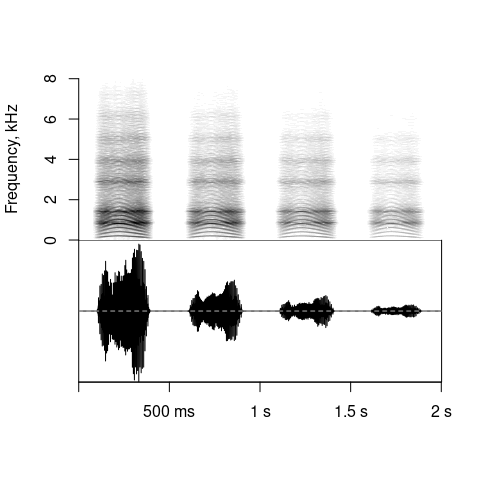The dynamic amplitude range is determined by `dynamicRange`. This parameter sets the minimum level of loudness, below which components are discarded as essentially silence. For maximum sound quality, set a high dynamicRange, like 120 dB. This helps to avoid artifacts like audibly clicking harmonics, but it also slows down sound generation. The default is 80 dB.

Rapid amplitude modulation imitating a trill is implemented by multiplying the synthesized waveform by a wave with adjustable `amType` (“sine” or “logistic”), shape `amShape` (logistic only), frequency `amFreq`, and amplitude `amDep`:

``````s027 = soundgen(
sylLen = 1000, formants = NA,
# set the depth of AM (0% = none, 100% = max)
amDep = c(0, 100),
# set AM frequency in Hz (vectorized)
amFreq = c(50, 25),
# set the shape: 0 = close to sine, -1 = notches, +1 = clicks
amShape = 0,
# asymmetrical attack: 20 ms at the beginning and 140 ms at the end
attackLen = c(20, 140),
plot = TRUE, heights = c(1, 1), ylim = c(0, 1), windowLength = 100,
play = playback)``````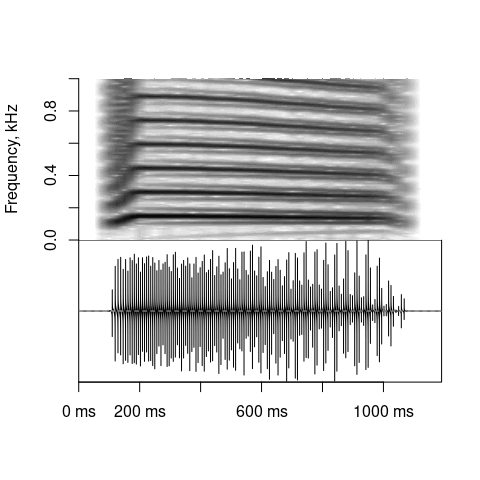A common special case of modifying the amplitude envelope of a synthesized or recorded sound is compression, which helps to make the amplitude remains relatively stable throughout the duration of the signal. There is a separate function for achieving this, namely `compressor()` AKA `flatEnv()`:

``````s = rnorm(500) * seq(1, 0, length.out = 500)
s1 = compressor(s, samplingRate = 1000, plot = TRUE,
killDC = TRUE, windowLength_points = 50)``````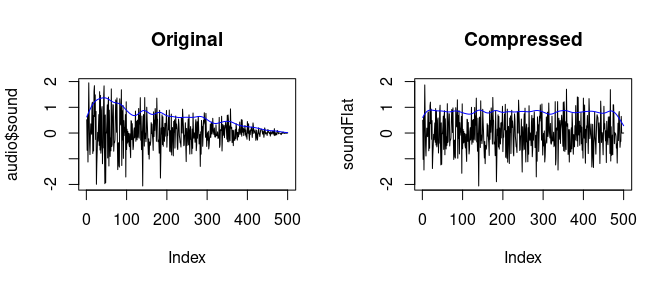Another common modification is to fade the sound in and/or out. One way to do this is to change the attack (which affects both the beginning and the end) or to use amplitude anchors. On other occasions, or if your sound already exists and you want to change it, the way to go about it is to use a separate function, `fade()`. This also gives you more options, e.g. different attack shapes, while soundgen() defaults to linear fade-in/out for attack.

``````# Create a sound with sharp attack
s028 = soundgen(sylLen = 300, pitch = 800, addSilence = 0, attackLen = 10)
# playme(s)``````
``````s029 = fade(s028, fadeIn = 50, fadeOut = 100, samplingRate = 16000,
shape = 'logistic', steepness = 1, plot = TRUE)
# playme(s029)
# different fades are available: linear, logarithmic, etc``````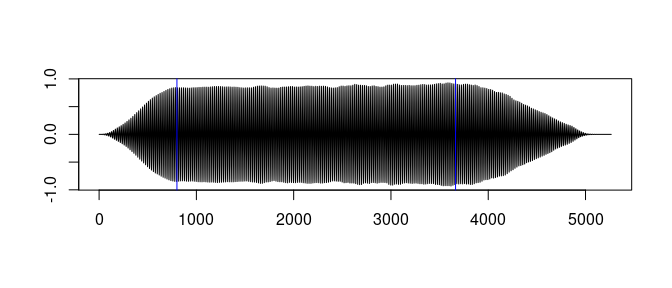TIP: attackLen in soundgen is applied only to voiced source, and before it is filtered (i.e., before formants are added). In case of artifacts increase attackLen or apply fade() after synthesizing the sound.

## 2.9 Spectral filter (formants)

### 2.9.1 Vowel presets

Argument `formants` (tab “Tract / Formants” in the app) sets the formants – frequency bands used to filter the excitation source. Just as an equalizer in a sound player amplifies some frequencies and dampens others, aappropriate filters can be applied to a tonal sound to make it resemble a human voice saying different vowels. Formants are created in the frequency domain using all-pole models if all formant amplitudes are positive and zero-pole models if there are anti-formants with negative amplitudes (Stevens, 2000, ch. 3).

Using presets for callers M1 and F1, you can directly specify a string of vowels. When you call `soundgen` with `formants = 'aouuuui'` or some such character string, the values are taken from `presets\$M1\$Formants` (or `presets\$F1\$Formants` if the speaker is “F1” in the Shiny app). Formants can remain the same throughout the vocalizations, or they can move. For example, `formants = 'ai'` produces a sound that goes smoothly from [a] to [i], while `formants = 'aaai'` produces mostly [a] with a rapid transition to [i] at the very end. Argument `formantStrength` (“Formant prominence” in the app) adjusts the overall effect of all formant filters at once, and `formantWidth` scales all bandwidths.

``s030 = soundgen(formants = 'ai', play = playback)``
``s031 = soundgen(formants = 'aaai', play = playback)``

### 2.9.2 Manual formants

Presets give you some rudimentary control over vowels. More subtle control is necessary for animal sounds, as well as for human vowels that are not included in the presets dictionary or for non-default speakers. For such cases you will have to specify at least the frequency of each formant (and optionally, also amplitude, bandwidth, and time stamps for each value). The easiest, and normally sufficient, approach is to specify frequencies only and have soundgen() figure out the appropriate amplitude and bandwidth for each formant. Bandwidth is calculated from frequency using a formula derived from human phonetic research. Namely, above 500 Hz it follows the original formula known as “TMF-1963” (Tappert, Martony, and Fant, 1963), and below 500 Hz it applies a correction to allow for energy losses at low frequencies (Khodai-Joopari & Clermont, 2002). Below 250 Hz the bandwidth starts to decrease again, in a purely empirical attempt to achieve reasonable values even for formant frequencies below ordinary human range. See the internal function soundgen:::getBandwidth() if you are interested and note that for anything but ordinary human voices it may be safer to specify formant bandwidths manually.

``````freqs = 2 ^ seq(log2(20), log2(20000), length.out = 500)
plot(freqs, soundgen:::getBandwidth(freqs), type = 'l',
log = 'xy', xlab = 'Center frequency, Hz',
ylab = 'Bandwidth, Hz',
main = 'Default formant bandwidths')
abline(v = 250, lty = 3)
abline(v = 500, lty = 3)``````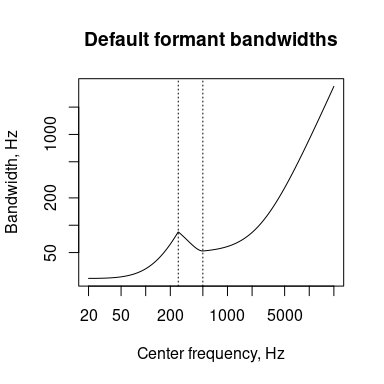Formant amplitudes are normally assumed to be determined by their frequency and bandwidth (see Stevens, 2000), but you can override this by specifying amplitudes explicitly. Note that changing the amplitude (or frequency, or bandwidth) of one formant affects the entire spectrum above it.

For moving formants, provide multiple values, which assumes equal time steps, or specify time points explicitly, where time varies from 0 to 1 (to be scaled appropriately depending on the length of sound). For example:

``````# shorthand specification with three stationary formants
formants = c(300, 2500, 3200)

# shorthand specification with two moving formants
formants = list(f1 = c(300, 900), f2 = c(2500, 1500))

# full specification with two moving formants and non-default amplitude and bandwidth
formants = list(
f1 = list(freq = c(300, 900),
amp = c(30, 10),
width = 120),
f2 = list(time = c(0, .2, 1),  # "time" is only needed for non-equidistant anchors
freq = c(2500, 2400, 1500),
amp = 30,
width = c(0, 220, 240)))``````

Feed these lists into soundgen() to hear what they sound like.

### 2.9.3 Vocal tract length

In addition to user-specified formants, higher formants are added automatically based on the length of vocal tract length estimated from the user-specified formant frequency values. The function that estimates vocal tract length is imaginatively called `estimateVTL`:

``estimateVTL(formants = c(400, 1800, 2550, 4100), plot = TRUE)  ``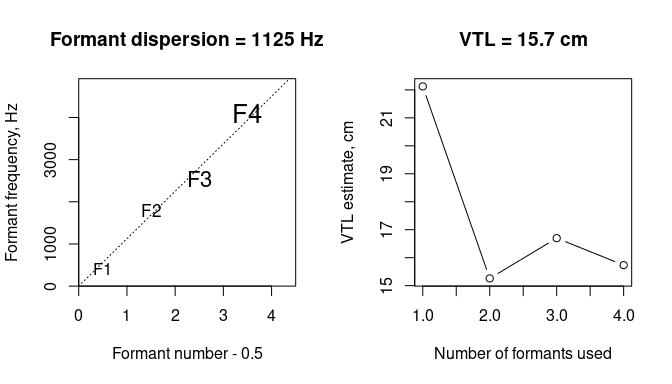``##  15.73333``
``# 17.5 cm``

TIP A more general function called `schwa()` both estimates VTL and allows you to compare measured formant frequencies with those expected for a neutral schwa sound and perform more sophisticated operations with formants. See `?schwa` for more details if you are working with vowels

It is usually useful to allow soundgen to create upper formants automatically based on the estimated VTL, since upper formants are typically less perceptually salient. As a result, we don’t want to spend too much time specifying them manually, but still we don’t want them to be completely absent, either, since that makes for very weak high frequencies in the spectrum. An approximation based on standard open-closed tube models is often good enough for calculating the missing formant frequencies. You can remove them by setting `temperature = 0` or `formantDepStoch = 0`, but note that without higher formants the entire spectrum loses energy at higher frequencies:

``s032 = soundgen(formants = c(800, 1200), play = playback, plot = TRUE)``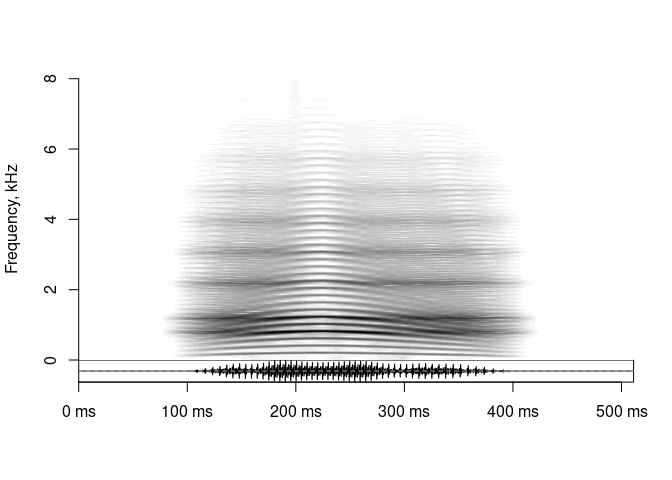``````s033 = soundgen(formants = c(800, 1200), formantDepStoch = 0,
play = playback, plot = TRUE)``````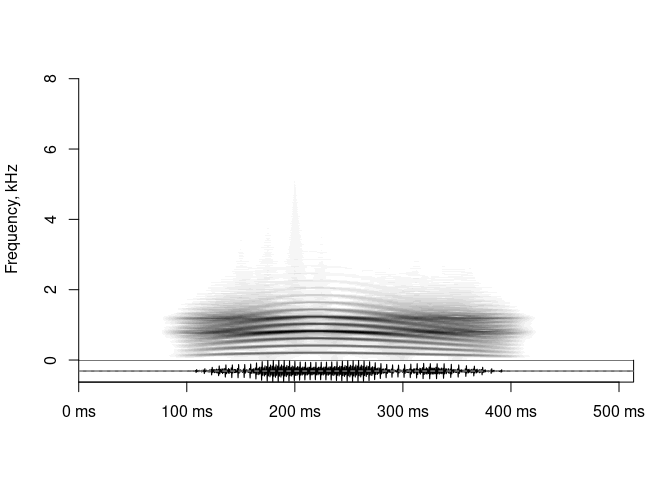Another useful method is to specify vocal tract length without any formants. In this case soundgen() approximates a neutral schwa sound for an animal with a vocal tract that looks like a uniform tube of this length. Crude, but often sufficient. A toy example (check presets for some more realistic sounds created using this method):

``````s034 = soundgen(
sylLen = 800, formants = NULL, rolloff = -6,
vocalTract = c(12, 18, 19), formantCeiling = 5,
play = playback, plot = TRUE)``````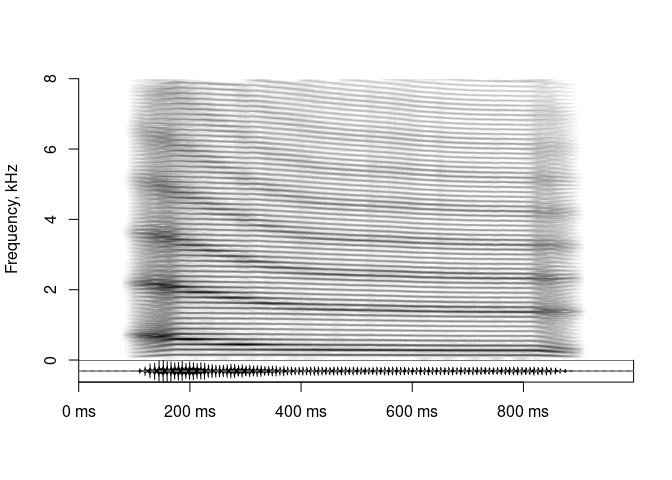For very long vocal tracts, it is advisable to increase `formantCeiling` to ~5-10 (i.e., 5 times the Nyquist frequency), otherwise the filter dampens high frequencies too much. The default setting is `formantCeiling = 2`, which is much faster and not too inaccurate for human-length vocal tracts. Note that vocal tract length can be variable, providing an easy way to create parallel formant transitions.

Unlike the argument “mouth”, variable “vocalTract” parameter recalculates formant bandwidths (unless these are specified manually) and is thus more accurate, but it requires you to specify a reasonable vocal tract length, in cm. If you provide both a few formant frequencies and a variable `vocalTract`, formants are synthesized as specified at the initial value of `vocalTract` (that is, at the very beginning of the sound) and can deviate afterwards as VTL changes. Careful with such combinations: make sure the initial value of the VTL is reasonable for these formant frequencies (any VTL is fine towards the end of the sound - the user-specified formants will move accordingly). Examples:

``````formants = list(f1 = c(500, 250), f2 = c(1500, 2800), f3 = 3500, f4 = 4300)
estimateVTL(formants)  # 13.7 cm - so the initial VTL should be close to 13.7``````
``##  13.58204``
``````s035 = soundgen(
sylLen = 800, rolloff = -6, formants = formants,
vocalTract = list(time = c(0, .3, 1), value = c(14, 28, 25)),
play = playback, plot = TRUE, main = 'Good initial VTL')``````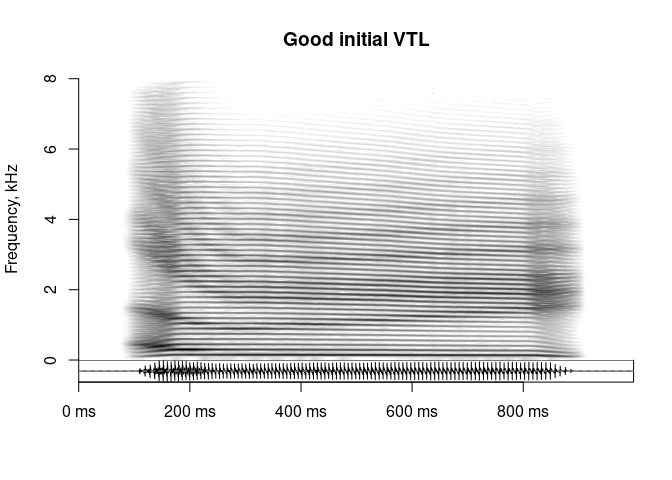``````# wrong: VTL too high for these formants, causing an overlap
s036 = soundgen(
sylLen = 800, rolloff = -6, formants = formants,
vocalTract = list(time = c(0, .3, 1), value = c(18, 28, 25)),
play = playback, plot = TRUE, main = 'Bad initial VTL')``````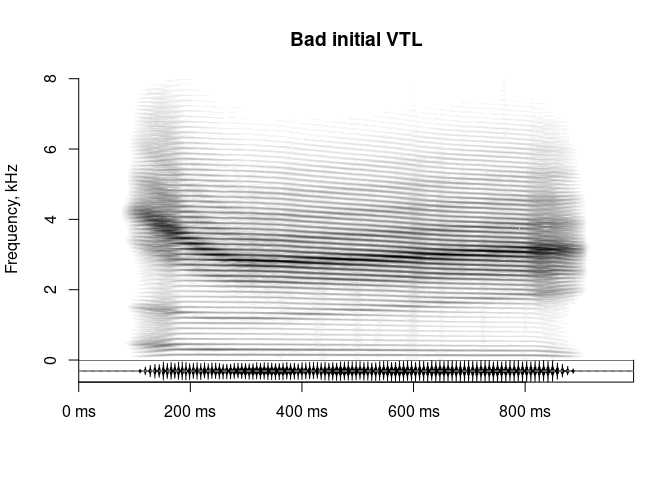### 2.9.4 Spectral envelope

Sometimes it may be useful to view moving formants before synthesizing the sound, or you may want to create a spectral envelope and then apply it to another sound with `transplantFormants()`. The way to create and view a spectral envelope is to call the function that handles all formant processing under the hood in soundgen, namely `getSpectralEnvelope`:

``````# plotting directly from getSpectralEnvelope() in spectrogram form
s = getSpectralEnvelope(nr = 1024,  # freq bins in FFT frame (window_length / 2)
nc = 50,    # time bins
samplingRate = 16000,
formants = list(f1 = c(500, 250),
f2 = c(1500, 2800),
f3 = 3500, f4 = 4300),
plot = TRUE,
dur = 1500,   # just an example
colorTheme = 'seewave',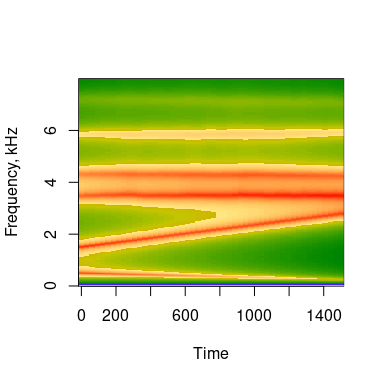Note that, in addition to formants, lip and nose radiation are also handled by this function (see the next section on mouth opening). This has the effect of boosting high frequencies.

TIP When using the app, you can start with a formant preset by typing in a vowel string, and then you can modify it. This way you don’t have to remember the right format. If you edit the list of formants and nothing in the sound seems to be changing, there may be a misprint, missing comma, etc.

### 2.9.5 Antiformants

For even more advanced spectral filters, you can specify both formants (poles) and antiformants (zeros). This may be useful if you want to create a nasalized sound. The numbering of formants is arbitrary, as long as they are arranged in the right order. For example, if you want to insert a new formant between F1 and F2 without renaming all higher formants, call it “f1.5” or something like that. It is important to use a non-integer number, since otherwise these additional formants will be inappropriately used to estimate the length of vocal tract and adding stochastic formants above the ones you specify (that is, if temperature > 0 and vocalTract = NA).

For example, a slow transition from [a] to [a nasalized] might be coded as follows (note that formant f1.7 has negative amplitude, so f1.5 and f1.7 form a pole-zero pair):

``````formants = list(
f1   = list(time = c(0, 1), freq = c(880, 900),
amp = c(25, 15), width = c(80, 120)),
f1.5 = list(time = c(0, 1), freq = 600,
amp = c(0, 15), width = 80),   # additional pole
f1.7 = list(time=c(0, 1), freq = 750,
amp = c(0, -15), width = 80),  # zero
f2   = list(time = c(0, 1), freq = c(1480, 1250),
amp = c(30, 20), width = c(120, 200)),
f3   = list(time=c(0, 1), freq = c(2900, 3100),
amp = 25, width = 200))
s037 = soundgen(sylLen = 1500, play = playback, pitch = 140, formants = formants)
spectrogram(s037, samplingRate = 16000, ylim = c(0, 4), contrast = .5,
windowLength = 10, step = 5, colorTheme = 'seewave')
# long-term average spectrum (less helpful for moving formants but very good for stationary):
# seewave::meanspec(s, f = 16000, wl = 256)  ``````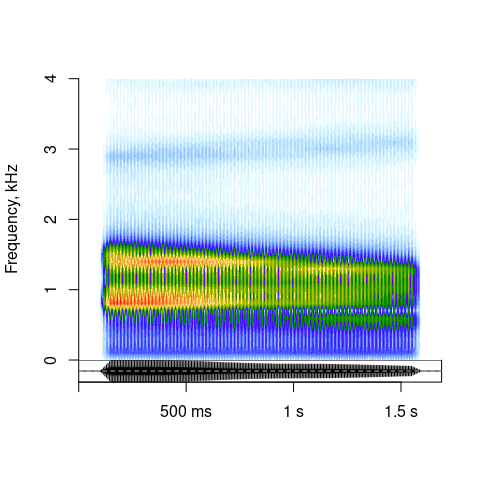If you look at the filter towards the end of the sound, you can observe the additional zero-pole pair between the first and second formant:

``````se = getSpectralEnvelope(nr = 512, nc = 100, formants = formants)
plot(as.numeric(rownames(se)), 20 * log10(se[, ncol(se)]),
type = 'l', xlab = 'KHz', ylab = 'dB')``````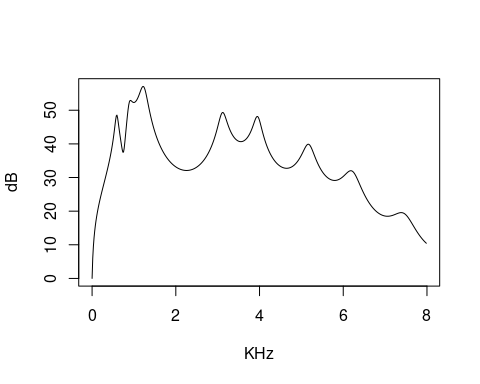### 2.9.6 Mouth opening

In addition to variable vocal tract length (`vocalTract` argument), an even easier shortcut for creating parallel formant transitions without coding all transitions by hand is provided by the `mouth` argument (in the app, tab “Tract / Mouth opening”). This can be thought of as a hyper-parameter offering an easy way to define moving formants within a bout (easy because you don’t need to know the VTL in cm): all formants simply go down relative to specified values as the mouth closes and rise as it opens (see Moore, 2016).

In addition, an open mouth has lip radiation, which has the effect of amplifying higher frequencies. Lip radiation is replaced by nose radiation when the mouth is completely closed, dampening the higher frequencies, and the vowel is automatically nasalized using a simple approximation (Hawkins & Stevens, 1985). Basically, with the mouth closed we switch from a tube open at one end to a tube closed at both ends and coupled with a (simplified) nasal cavity. Despite being a crude model of what really happens when a vocalizing animal closes its mouth, in many cases `mouth` can save you a lot of manual coding of formants. Here is a simple example, with the mouth gradually opening and closing again:

``````s038 = soundgen(sylLen = 1200, play = playback, pitch = 140,
mouth = list(time = c(0, .3, .75, 1),
value = c(0, 0, .7, 0)))
spectrogram(s038, samplingRate = 16000,
ylim = c(0, 4), contrast = .5,
windowLength = 10, step = 5,
colorTheme = 'seewave')``````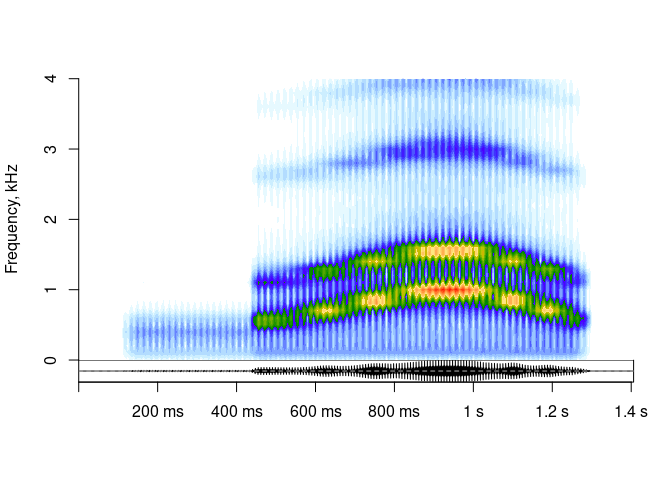TIP Here and elsewhere, I talk about applying soundgen to the task of synthesizing non-human sounds. It does work, but be aware that many computational routines are based on human phonetic research, simply because there is vastly more data available on human vocal production. For example, formant bandwidths and spectral consequences of nasalization are estimated based on human phonetics, but it is far from clear to what extent these equations are applicable to sounds produced by non-human mammals. Bird calls are again a whole new ball game. And once you move on to insects or non-biological sounds, just forget about hyperparameters like `mouth` and code everything at the lowest possible level.

### 2.9.7 Formant locking

The standard source-filter model (Fant, 1971) assumes that the vibration of the vocal folds is independent of the configuration of the supraglottal vocal tract - that is, that the source and filter are independent. However, in some situations this assumption does not hold, and the filter can have a noticeable effect on the vibration of the vocal folds. An example of such source-filter interaction that is implemented in soundgen is formant locking, in which the fundamental frequency or a higher harmonic becomes temporarily “locked” to the frequency of a formant. The relevant parameter is `formantLocking` (0 = none, 1 = the entire sound, vector form also accepted). See the internal function `soundgen:::lockToFormants()` for more information and examples.

In humans, spontaneous formant locking is usually observed in high-pitched sounds like screams, although formant matching can also be performed intentionally in order to maximize sonority, as in soprano singing, or to produce unusual vocal effects such as Tuvinian throat singing. In animal vocalizations, formant locking appears to be particularly common when the vocal tract is long and formants are closely spaced (my speculation). For example, elk bugles often contain a series of stepwise pitch jumps from one formant to the next, a bit like this:

``````s039 = soundgen(
sylLen = 1500, rolloff = -20,
pitch = c(500, 1000, 1800, 1700, 500),
formants = NULL, vocalTract = 55,
formantLocking = c(0, 1, 1, 1, 1, 0),  # except the beginning and end
shortestEpoch = 200,  # affects both subharmonics (if any) and formantLocking
noise = -20,  # just to make formants visible in this example
temperature = .1,
samplingRate = 22000, pitchSamplingRate = 22000,
play = playback, plot = TRUE, ylim = c(0, 8)
)``````